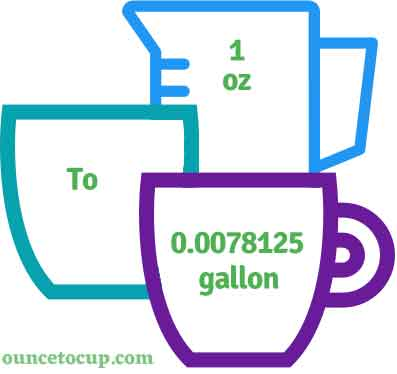# Ounce to Gallon (oz to gal conversion)

Are you cooking your favorite dish? The detailed chart in the recipe includes the calculation of 1 ounces to gal conversion? Do not worry; check this conversion tool to find how many 1 ounces equal to gal in a minute. This 1 oz to gal converter gives an exact measurement for any recipe you prepare.

Ounce Value:

oz

Gallon Value:

gal

1 Ounce = 0.0078125 Gallon
(1 oz = 0.0078125 gal)

Try our auto 1 ounce to gallon calculator (Without Convert Button), Just change the first field value and you got final value.## How many gal is a oz?

We know that the volume value of 1 oz is equal to 0.0078125 gal. If you want to convert 1 fluid oz to an equal number of gal, just divide the volume value by 128. Hence, 1 Ounce is equal to 0.0078125 gal.

The Answer is: 1 US Fluid Ounces = 0.0078125 US gal

1 oz = 0.0078125 gal

Many of them try to search or find an answer for what is 1 ounces in gal? So, we’ll start with 1 fl to oz gal conversion to know how big is 1 oz.

## How To Calculate 1 fluid oz to gal?

To calculate 1 fluid ounces to an equal number of gallon, simply follow the steps below.

Fluid Ounces to Gallons formula is:

Gallon = Fluid Ounce / 128

Assume that we are finding out how many gal were found in 1 fl oz of water, divide by 128 to get the result.

Applying to Formula: gal = 1 oz / 128 = 0.0078125 gal.

## How To Convert oz to gal?

• To convert fluid ounces to gal,
• Simply divide the fluid ounce value by 128.
• Applying to the formula, gal = 1 ounces / 128 [1/128].
• Hence, 1 ounces is equal to 0.0078125 gal.

## Some quick table references for fluid ounce to gallon conversions:

Ounce [oz]Gallon [gal]
1 oz0.0078125 gal
2 oz0.0156 gal
3 oz0.0234 gal
4 oz0.0313 gal
5 oz0.0391 gal
6 oz0.0469 gal
7 oz0.0547 gal
8 oz0.0625 gal
9 oz0.0703 gal
10 oz0.0781 gal
11 oz0.0859 gal
12 oz0.0938 gal
13 oz0.1016 gal
14 oz0.1094 gal
15 oz0.1172 gal

## Reverse Calculation: How many ounces are in a gal?

• To convert 1 gal to oz,
• Simply multiply the 1 gal by 128.
• Then, applying the formula, ounce = 1 gal * 128 [1x128 = 128].
• Hence, 1 gal is equal to 128 oz.

### Related Converter:

Formula: Ounce to gal

gal = ounce / 128

Applying to Formula,

gal = 1/128 = 0.0078125

1 oz = 0.0078125 gal